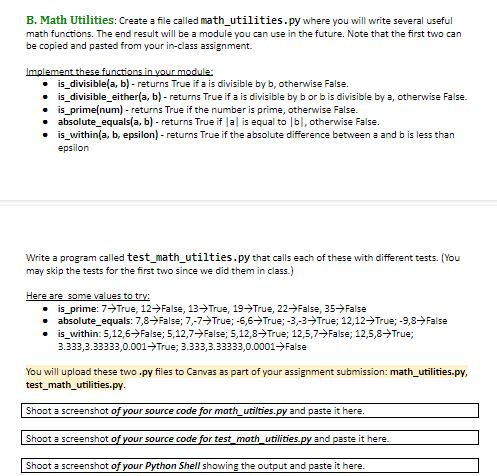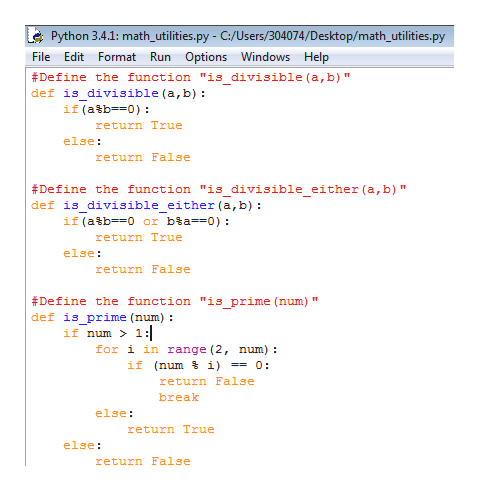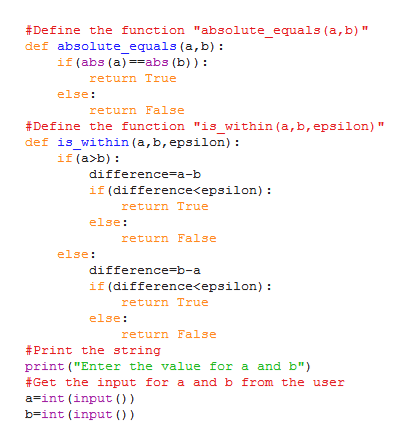# B. Math Utilities: Create a file called math_utilities.py where you will write several usefulmath functions. The end result will be a module you can use in the future. Note that the first two canbe copied and pasted from your in-class assignmentImplement these functions in vour moduleis_divisible(a, b)- returns True if a is divisible by b, otherwise False.is_divisible_either(a, b)- returns True if a is divisible by b or bis divisible by a, otherwise False.is prime(num) - returns True if the number is prime, otherwise False.absolute_equals(a, b) - returns True if Ja is equal to lb, otherwise Falseis_within(a, b, epsilon)- returns True if the absolute difference between a and b is less thanepsilonWrite a program called test math_utilties.py that calls each of these with different tests. (Youmay skip the tests for the first two since we did them in class.)Here are some values to tryis_prime: 7-True, 12- False , 13- True, 19 True, 22 False, 35- Falseabsolute_equals: 7,8- False; 7,-7True; -6,6 True; -3,-3-True; 12,12- True; -9,8-Falseis_within: 5,12,6-False; 5,12,7-False; 5,12,8True; 12,5,7- False; 12,5,8True;3.333,3.33333,0.001 True; 3.333,3.33333,0.0001FalseYou will upload these two .py files to Canvas as part of your assignment submission: math utilities.py,test math_utilities.py.Shoot a screenshot of your source code for math_utilties.py and paste it hereShoot a screenshot of your source code for test_math_utilities.py and paste it here.Shoot a screenshot of your Python Shell showing the output and paste it here.

Question
2 views

////////////////

////////////////

////////////////

////////////////help_outlineImage TranscriptioncloseB. Math Utilities: Create a file called math_utilities.py where you will write several useful math functions. The end result will be a module you can use in the future. Note that the first two can be copied and pasted from your in-class assignment Implement these functions in vour module is_divisible(a, b)- returns True if a is divisible by b, otherwise False. is_divisible_either(a, b)- returns True if a is divisible by b or bis divisible by a, otherwise False. is prime(num) - returns True if the number is prime, otherwise False. absolute_equals(a, b) - returns True if Ja is equal to lb, otherwise False is_within(a, b, epsilon)- returns True if the absolute difference between a and b is less than epsilon Write a program called test math_utilties.py that calls each of these with different tests. (You may skip the tests for the first two since we did them in class.) Here are some values to try is_prime: 7-True, 12- False , 13- True, 19 True, 22 False, 35- False absolute_equals: 7,8- False; 7,-7True; -6,6 True; -3,-3-True; 12,12- True; -9,8-False is_within: 5,12,6-False; 5,12,7-False; 5,12,8True; 12,5,7- False; 12,5,8True; 3.333,3.33333,0.001 True; 3.333,3.33333,0.0001False You will upload these two .py files to Canvas as part of your assignment submission: math utilities.py, test math_utilities.py. Shoot a screenshot of your source code for math_utilties.py and paste it here Shoot a screenshot of your source code for test_math_utilities.py and paste it here. Shoot a screenshot of your Python Shell showing the output and paste it here. fullscreen
check_circle

Step 1

A python program to display the boolean values for the given functions is provided below;

Program code:

File name: “math_utilities.py

#Define the function "is_divisible(a,b)"

def is_divisible(a,b):

if(a%b==0):

return True

else:

return False

#Define the function "is_divisible_either(a,b)"

def is_divisible_either(a,b):

if(a%b==0 or b%a==0):

return True

else:

return False

#Define the function "is_prime(num)"

def is_prime(num):

if num > 1:

for i in range(2, num):

if (num % i) == 0:

return False

break

else:

return True

else:

return False

#Define the function "absolute_equals(a,b)"

def absolute_equals(a,b):

if(abs(a)==abs(b)):

return True

else:

return False

#Define the function "is_within(a,b,epsilon)"

def is_within(a,b,epsilon):

if(a>b):

difference=a-b

if(difference<epsilon):

return True

else:

return False

else:

difference=b-a

if(difference<epsilon):

return True

else:

return False

#Print the string

print("Enter the value for a and b")

#Get the input for a and b from the user

a=int(input())

b=int(input())

#Print the boolean value return from the function "is_divisible(a,b)"

print ("is_divisible(",a,",",b,"): ",is_divisible(a,b))

#Print the boolean value return from the function "is_divisible_either(a,b)"

print ("is_divisible_either(",a,",",b,"): ",is_divisible_either(a,b))

#Print the boolean value return from the function "absolute_equals(a,b)"

print ("absolute_equals(",a,",",b,"): ",absolute_equals(a,b))

#Print the string

print ("Enter the number to find prime")

#Get the input to check prime number

num=int(input())

#Print the boolean value return from the function "is_prime(num)"

print ("is_prime(",num,"): ",is_prime(num))

#Print the string

print ("Enter the value for epsilon")

#Get the input for epsilon

epsilon=int(input())

#Print the boolean value return from the function "is_within(a,b,epsilon)"

print ("is_within(",a,",",b,",",epsilon,"): ",is_within(a,b,epsilon))

Step 2

Explanation:

• The functions given in the question have been defined with the keyword “def”.
• The statements inside the body are provided with the proper indentation and syntax.
• The inputs to perform the given functions are get from the user through keyboard using “input()” function.
• The appropriate function is called and the result is printed using “print” statement.

Screenshot of program #1:help_outlineImage TranscriptionclosePython 3.4.1: math_utilities.py - C:/Users/304074/Deskto p/math_utilities.py File Edit Format Run Options Windows Help #Define the function "is divisible (a,b)" def is divisible (a,b) if (a%b0) return True else: return False #Define the function "is divisible either (a,b)" def is divisible either (a,b) if (atb0 or bla-=0) return True else: return False #Define the function "is prime (num) def is_prime (num) : if num > 1: for i in range (2, num): if (num i 0: return False break else return True else: return False fullscreen
Step 3

Screenshot of program #2...help_outlineImage TranscriptioncloseDefine the function "absolute_equals (a,b)" def absolute_equals (a,b) if (abs (a)abs (b)) return True else: return False Define the function "is_within (a, b, epsilon) def is within (a,b, epsilon) if (a>b) difference-a-b if (difference

### Want to see the full answer?

See Solution

#### Want to see this answer and more?

Solutions are written by subject experts who are available 24/7. Questions are typically answered within 1 hour.*

See Solution
*Response times may vary by subject and question.
Tagged in

### Python